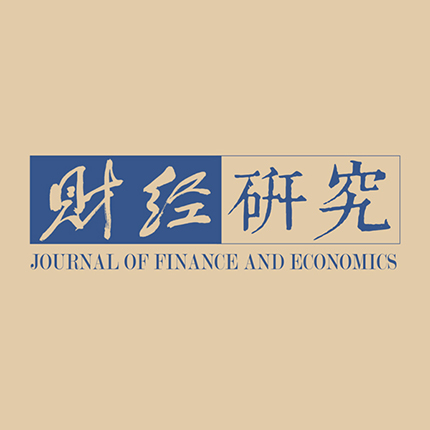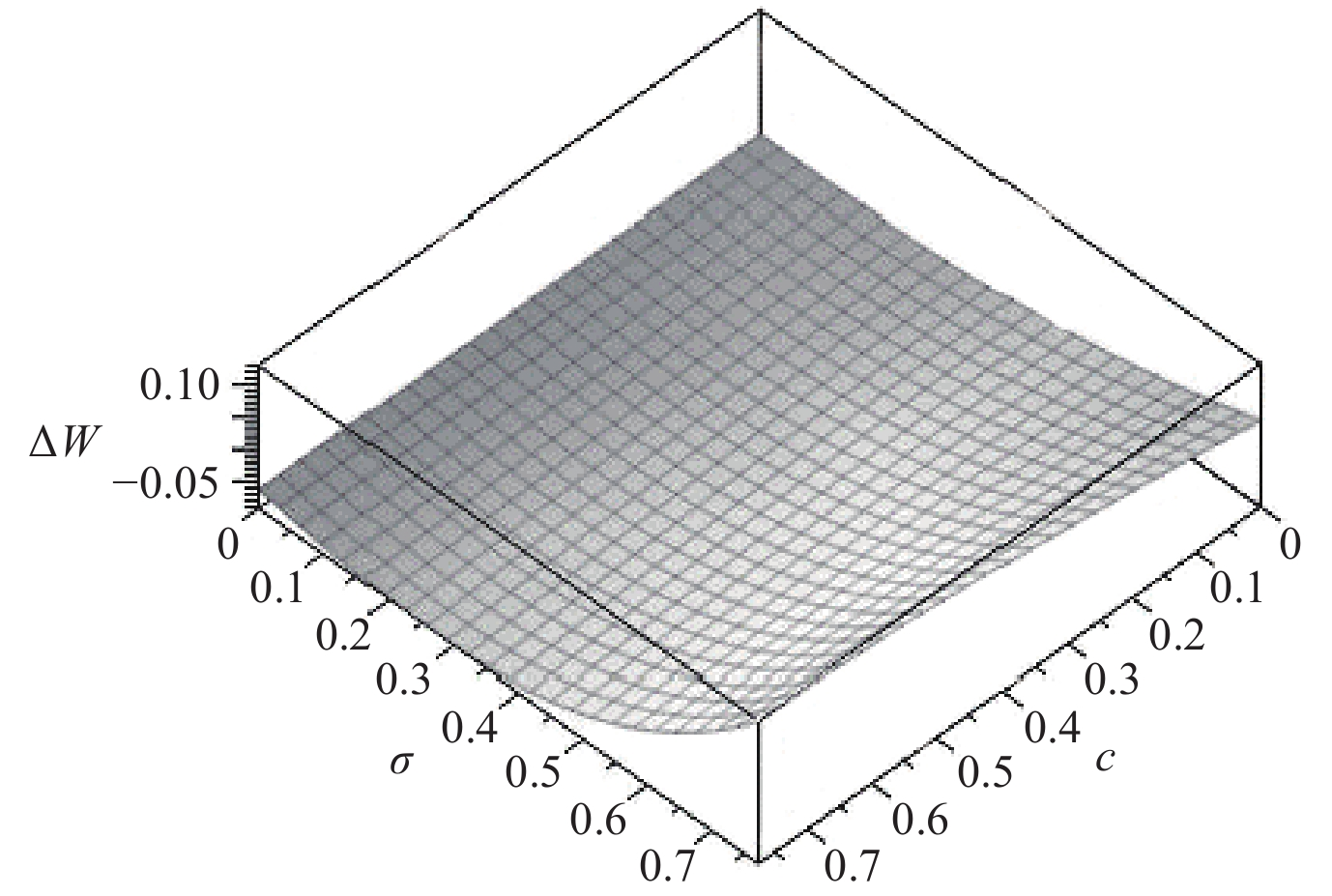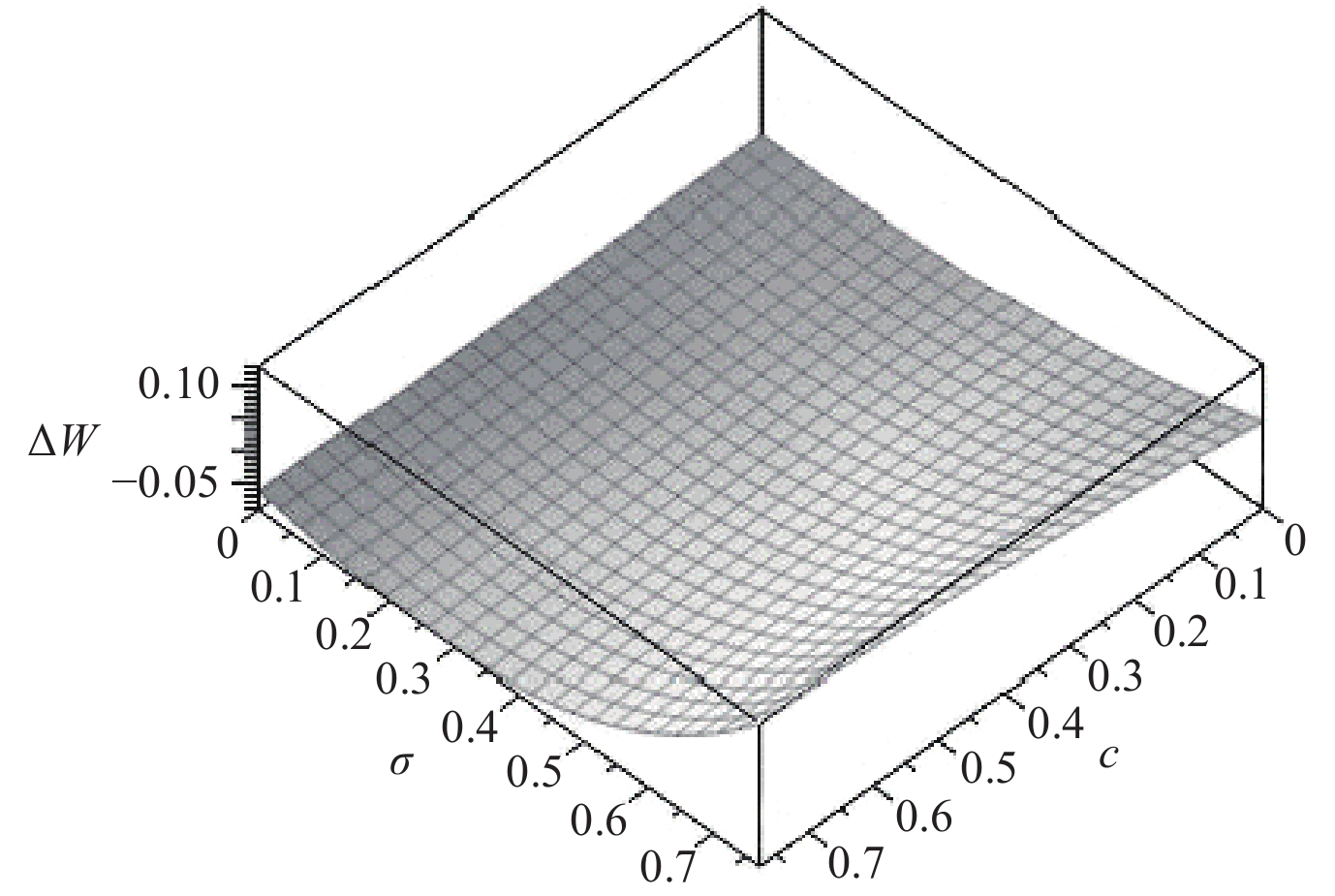﻿ “店选网购”与“店网并购”的二重性及社会福利效应<sup>*</sup>《财经研究》
2023第49卷第4期
“店选网购”与“店网并购”的二重性及社会福利效应*

1. 南京财经大学 国际经贸学院，江苏 南京 210023;
2. 天津财经大学 商学院，天津 300222

The Duality and Social Welfare Effects of “Showrooming” and “Online-Offline Merger”
Xu Honghai1, Liu Chan2
1. School of International Economics and Trade, Nanjing University of Finance & Economics, Nanjing 210023, China;
2. School of Business, Tianjin University of Finance & Economics, Tianjin 300222, China
Summary: With the rise of the Internet and E-commence, “showrooming” widely emerges, a pattern such that acquire service and information about products at offline stores but purchase them online. Different from “direct online shopping”, showrooming can promote competition on the one hand, and on the other hand, it may cause free-rider problems. The increasing competition between online and offline stores has encouraged them to realize the integration of New Retail through mergers, which has gradually become a new development trend. “Online-offline merger” has the negative effect of restricting competition, but in the case of showrooming, it also has the positive effect of alleviating the free-rider. How to balance the relationship between the efficiency improvement in solving the free-rider and the effect of restricting competition, and how to explore the social welfare effects are the key problems to be solved to analyze the competition impact of online-offline merger. This paper analyzes the welfare effects of showrooming and online-offline merger by constructing the Hotelling competition model. The conclusion shows that: Showrooming can reduce the prices and profits of online and offline stores, but increase consumer surplus. The impact on social welfare depends on the probability of buying desirable goods. The online-offline merger will indeed increase the prices and profits of online and offline stores, and the impact on consumer surplus and social welfare depends on the probability of buying desirable goods. To sum up: (1) When the probability is high, showrooming will improve social welfare, while online-offline merger will damage social welfare. (2) When the probability is low, showrooming will damage social welfare, while online-offline merger will improve social welfare. (3) When the probability is in the middle level, the social welfare effects of the two are uncertain. Accordingly, the anti-monopoly authority can make corresponding approval or prohibition decisions on online-offline merger. The marginal contributions of this paper are as follows: (1) By distinguishing the duality of showrooming, and focusing on the analysis of its welfare effects, it enriches the economic research on showrooming. (2) It explores the effectiveness of online-offline merger in dealing with the negative impact of showrooming and provides more strategic choices for relevant enterprises to solve channel conflicts. (3) By analyzing the social welfare effects of online-offline merger in the case of showrooming, it provides an economic theoretical basis for the implementation of public policies related to online-offline merger.
Key words: showrooming    online-offline merger    free-rider    social welfare    anti-monopoly

（一）假设条件图 1 消费者购物决策与效用
 $\left\{ \begin{gathered} E\left( {{U_s}} \right) = \sigma \left( {V - {P_s} - tX} \right) - \left( {1 - \sigma } \right)tX = \sigma \left( {V - {P_s}} \right) - tX \\ E\left( {{U_{so}}} \right) = \sigma \left[ {V - {P_o} - t\left( {1 - X} \right) - tX} \right] - \left( {1 - \sigma } \right)tX = \sigma \left[ {V - {P_o} - t\left( {1 - X} \right)} \right] - tX \\ E\left( {{U_o}} \right) = \sigma \left[ {V - {P_o} - t\left( {1 - X} \right)} \right] - \left( {1 - \sigma } \right)\left[ {{P_o} + t\left( {1 - X} \right)} \right] = \sigma V - {P_o} - t\left( {1 - X} \right) \\ \end{gathered} \right.$ (1)

（二）基准情形：“店网分离”结构下无“店选网购”情形的均衡结果

 ${Q}_{s}^{n}=\sigma {X}^{n}\text{，}{Q}_{o}^{n}=1-{X}^{n}$ (2)

 ${\pi }_{s}^{n}=\left({P}_{s}^{n}-c\right){Q}_{s}^{n}\text{，}{\pi }_{o}^{n}={P}_{o}^{n}{Q}_{o}^{n}$ (3)

 ${P}_{s}^{N}=\frac{3t+2\sigma c}{3\sigma }\text{，}{Q}_{s}^{N}=\frac{\sigma \left(3t-\sigma c\right)}{6t}\text{，}{\pi }_{s}^{N}=\frac{{\left(3t-\sigma c\right)}^{2}}{18t}$ (4)
 ${P}_{o}^{N}=\frac{3t+\sigma c}{3}\text{，}{Q}_{o}^{N}=\frac{3t+\sigma c}{6t}\text{，}{\pi }_{o}^{N}=\frac{{\left(3t+\sigma c\right)}^{2}}{18t}$ (5)

 $C{S^N} = \int_0^{{X^N}} {E\left( {{U_s}} \right){\rm{d}}{X}} + \int_{{X^N}}^1 {E {({U_o})} {\rm{d}}{X}} = \sigma V + \frac{{{c^2}{\sigma ^2} - 18ct\sigma - 45{t^2}}}{{36t}}$ (6)

（一）“店网分离”结构下有“店选网购”情形的均衡结果

 ${Q}_{s}=\sigma {X}_{1}\text{，}{Q}_{so}=\sigma \left({X}_{2}-{X}_{1}\right)\text{，}{Q}_{o}=\left(1-{X}_{2}\right)$ (7)

 ${\pi }_{s}=\left({P}_{s}-c\right){Q}_{s}-c{Q}_{so}\text{，}{\pi }_{o}={P}_{o}\left({Q}_{so}+{Q}_{o}\right)$ (8)

 ${P}_{s}^{*}=\frac{\left(3-\sigma \right)t}{\tau }\text{，}{Q}_{s}^{*}=\frac{\sigma \left(1-\sigma +{\sigma }^{2}\right)}{\tau }\text{，}{\pi }_{s}^{*}=\frac{\sigma {\left(3-\sigma \right)}^{2}t}{{\tau }^{2}}-\frac{2\sigma \left(1-\sigma \right)\left(3-\sigma \right)c}{\tau \left(2-\sigma \right)}$ (9)
 ${P}_{o}^{*}=\frac{\left(2-{\sigma }^{2}\right)t}{\tau }\text{，}{Q}_{o}^{*}=\frac{2+4\sigma -3{\sigma }^{2}+2{\sigma }^{3}-{\sigma }^{4}}{\tau \left(2-\sigma \right)}\text{，}{\pi }_{o}^{*}=\frac{{\left(2-{\sigma }^{2}\right)}^{2}t}{\tau \left(2-\sigma \right)}$ (10)

 $\begin{gathered} C{S^*} = \int_0^{X_1^*} {E\left( {{U_s}} \right){\rm{d}}X} + \int_{X_1^*}^{X_2^*} {E\left( {{U_{so}}} \right)} {\rm{d}}X + \int_{X_2^*}^1 {E\left( {{U_o}} \right){\rm{d}}X} \\ = \sigma V + \frac{{\left( {{\sigma ^6} - 3{\sigma ^5} + {\sigma ^4} - 8{\sigma ^3} + 31{\sigma ^2} - 6\sigma - 28} \right)t}}{{2{\tau ^2}\left( {2 - \sigma } \right)}} \\ \end{gathered}$ (11)

（二）有无“店选网购”情形的均衡结果比较静态分析

1. “店选网购”对店商和网商的影响

2. “店选网购”对消费者的影响

“店选网购”对消费者剩余的影响可以根据有无“店选网购”情形下消费者购物方式的转变情况，分为三个部分来讨论：（1）对于有无“店选网购”情形下始终选择“店选店购”和“直接网购”的消费者，店商和网商价格降低无疑会提高其效用水平。（2）在“店选网购”的竞争作用下，原来选择“店选店购”的部分消费者可能会转为“店选网购”或“直接网购”。转为“店选网购”的消费者既能获得店选服务，又可从低价网购中受益；转为“直接网购”的消费者虽不能获得店选服务，却可从低价网购中获得更多收益，所以这两部分消费者的效用水平都会有所提升。（3）在“店选网购”影响下，原来选择“直接网购”的消费者可能会转为选择“店选网购”，同时享有店商服务和网商低价，也可能在店选过程中发现商品不合意而放弃购买，从而避免因为购买到不合意商品而遭受的损失，这些都会提高此部分消费者剩余水平。总起来看，尽管“店选网购”因存在网商搭店商便车的问题而直接损害店商利益，但是作为消费者基于经济理性的选择，当然会为其带来一定的收益。“店选网购”促进店商和网商价格竞争，并且店选能够满足试选需求并在一定程度上避免购买到不合意商品带来的损失，因而会提高消费者剩余。

3. “店选网购”对社会福利的影响

$\Delta W = {W^*} - {W^N} =( \pi _s^* + \pi _o^* + C{S^*} ) - \left( {\pi _s^N + \pi _o^N + C{S^N}} \right)$
，后续的进一步分析需分四种情况讨论。（1）当 $0 < \sigma < 0.597$ 时，恒有 ${W^*} < {W^N}$ ，此时“店选网购”会降低社会福利。（2）当 $0.597 < \sigma < 0.609$ 时， ${W^*} - {W^N}$ 的正负值主要取决于服务成本 $c$ ：若 $0 < c < { A}$ ，则有 ${W^*} < {W^N}$ ，也就是说“店选网购”会降低社会福利；若 ${ A} < c < { B}$ ，则有 ${W^*} > {W^N}$ ，即“店选网购”会提高社会福利；若 ${ B} < c < {{\left( {3 - \sigma } \right)t} \mathord{\left/ {\vphantom {{\left( {3 - \sigma } \right)t} \tau }} \right. } \tau }$ ，则有 ${W^*} < {W^N}$ ，即“店选网购”会降低社会福利。（3）当 $0.609 < \sigma < 0.627$ 时， ${W^*} - {W^N}$ 的正负值同样也主要取决于服务成本 $c$ ：若 $0 < c < { A}$ ，则 ${W^*} < {W^N}$ ，即“店选网购”会降低社会福利；若 ${ A} < c < {{\left( {3 - \sigma } \right)t} \mathord{\left/ {\vphantom {{\left( {3 - \sigma } \right)t} \tau }} \right. } \tau }$ ，则 ${W^*} > {W^N}$ ，即“店选网购”会提高社会福利。（4）当 $0.627 < \sigma < 0.772$ 时，恒有 ${W^*} > {W^N}$ ，即此时“店选网购”会提高社会福利。为了直观地比较有无“店选网购”情形下社会福利结果的变化情况，我们将 $t$ 赋值为1，可得 $\Delta W$ $\sigma$ $c$ 的相互关系如图2所示。综上，本文提出命题3：“店选网购”对社会福利的影响取决于购买到合意商品的概率 $\sigma$ 。当 $\sigma$ 较小时，“店选网购”会降低社会福利；相反，当 $\sigma$ 较大时，“店选网购”会提高社会福利；而当 $\sigma$ 处于中间水平时，“店选网购”对社会福利的影响是不确定的，结果需要根据服务成本水平具体分析。图 2 有无“店选网购”情形的社会福利比较

“店选网购”对社会福利的影响主要取决于购买到合意商品的概率，其中的经济学逻辑在于购买到合意商品的概率会对消费者购物行为和企业的需求量产生影响，进而会对消费者剩余及店商和网商利润（生产者剩余）产生不同程度的影响。当购买到合意商品的概率较低时，一方面，消费者会倾向于先店选后再决定是否购买，这会耗费更多的购物成本；另一方面，较低的合意概率也意味着消费者更可能因为购买不到合意的商品而遭受经济损失。因此，尽管“店选网购”促进价格竞争的作用会提高消费者剩余，但提高幅度并不大。对于店商和网商而言，购买到合意商品概率较低的直接结果就是导致店商和网商的需求量和利润大幅下降。此时，“店选网购”对消费者和生产者剩余的综合结果则表现为“店选网购”会降低社会福利水平。相反，当购买到合意商品的概率较高时，“店选网购”能够相对较大幅度地提高消费者剩余，并且足以弥补“店选网购”对店商和网商带来的利润损失，综合结果表现为社会福利水平上升。当购买到合意商品概率居于中间水平时的结果比较复杂，“店选网购”对消费者剩余的提高幅度与店商和网商利润的降低幅度大致相当，最终可能提高也可能降低社会福利，需要根据具体情况具体分析。

（一）“店选网购”情形下店商和网商实行“店网并购”的均衡结果

 ${\pi ^i}{\text{ = }}\left( {P_s^i - c} \right)Q_s^i + \left( {P_o^i - c} \right)Q_{so}^i + P_o^iQ_o^i$ (12)

 ${P}_{s}^{I}=\frac{\left(2-\sigma \right)t-\sigma \left(1-\sigma \right)c}{2{\left(1-\sigma \right)}^{2}}\text{，}{Q}_{s}^{I}=\frac{\sigma }{2}$ (13)
 ${P}_{o}^{I}=\frac{\left(1+\sigma -{\sigma }^{2}\right)t-\sigma \left(1-\sigma \right)c}{2{\left(1-\sigma \right)}^{2}}\text{，}{Q}_{o}^{I}=\frac{\left(1-\sigma \right)\left(t+\sigma c\right)}{2\left(2-\sigma \right)t}$ (14)
 ${\pi ^I} = \frac{{{c^2}{{\left( {1 - \sigma } \right)}^2}{\sigma ^2} + 2ct\sigma \left( {{\sigma ^3} - 4{\sigma ^2} + 6\sigma - 3} \right) + {t^2}\left( {2{\sigma ^3} - 6{\sigma ^2} + 4\sigma + 1} \right)}}{{4t\left( {2 - \sigma } \right){{\left( {1 - \sigma } \right)}^2}}}$ (15)

“店网并购”后的消费者剩余也包括“店选店购”“店选网购”和“直接网购”三部分消费者剩余，市场均衡时的消费者剩余为：

 $\begin{gathered} C{S^I} = \int_0^{X_1^I} {E\left( {{U_s}} \right){\rm{d}}X} + \int_{X_1^I}^{X_2^I} {E\left( {{U_{so}}} \right)} {\rm{d}}X + \int_{X_2^I}^1 {E\left( {{U_o}} \right){\rm{d}}X} \\ = \sigma V + \frac{{{c^2}{\sigma ^2}{{\left( {1 - \sigma } \right)}^2} + 2ct\sigma \left( {1 - \sigma } \right)\left( {1 + \sigma - {\sigma ^2}} \right) - {t^2}\left( {2{\sigma ^3} - 6{\sigma ^2} + 7} \right)}}{{8t\left( {2 - \sigma } \right){{\left( {1 - \sigma } \right)}^2}}} \\ \end{gathered}$ (16)

（二）“店选网购”与“店网并购”情形的均衡结果比较静态分析

“店选网购”可以促进店商和网商之间的竞争，同时也有网商搭店商便车的问题。“店网并购”是将搭便车问题内部化的策略之一，却会限制店商和网商之间的竞争。那么，在“店选网购”情境下，“店网并购”究竟会对市场相关主体以及社会福利产生怎样的影响，这里通过比较分析“店网分离”结构下“店选网购”情形与“店选网购”情境下“店网并购”情形的均衡结果来揭示这一问题。

1. “店网并购”对店商和网商的影响

$Q_s^I + Q_{so}^I + Q_o^I < Q_s^* + Q_{so}^* + Q_o^*$
），表明“店网并购”提升了企业市场势力，一体化企业能够通过“限产提价”获得更多利润。此外，“店网并购”可以提高店商和网商利润的另一个主要原因在于，其可以缓解“店选网购”中搭便车问题的负面影响。尽管“店网并购”后仍有“店选网购”现象和网商搭店商便车的问题，但是店商为“店选网购”消费者提供服务所付出的成本可以从网商的收益中获得补偿，店商和网商不必再为争取“店选网购”消费者而竞相降价。

2. “店网并购”对消费者的影响

${ M} < c < {{t\left( {3 - \sigma } \right)} \mathord{\left/ {\vphantom {{t\left( {3 - \sigma } \right)} \tau }} \right. } \tau }$
，则有 ${\text{C}}{{\text{S}}^I} > C{S^*}$ ，即“店网并购”会提高消费者剩余。（3）当 $\sigma > 0.443$ 时，恒有 ${CS ^I} < C{S^*}$ ，即“店网并购”会降低消费者剩余。综合以上几种讨论结果，本文提出命题5：存在“店选网购”情况下，“店网并购”对消费者剩余的影响主要取决于购买到合意商品的概率 $\sigma$ 。当 $\sigma$ 较小时，“店网并购”可以提高消费者剩余；当 $\sigma$ 较大时，“店网并购”会降低消费者剩余；而当 $\sigma$ 处于中间水平时，“店网并购”对消费者剩余的影响结果是不确定的，具体结果需要根据服务成本水平具体分析。

3. “店网并购”对社会福利的影响图 3 “店网并购”与“店选网购”情形的社会福利比较

“店网并购”对社会福利与其对消费者剩余的影响规律和内在逻辑基本是一致的。当购买到合意商品的概率较小时，“店网并购”能够通过消减购物成本或购买到不合意商品的损失来提高消费者剩余，同时可以通过弱化店商与网商之间的价格竞争和缓解搭便车问题来改善生产者剩余，其最终结果自然会提高社会福利。相反，当购买到合意商品的概率较大时，价格上涨对消费者剩余的损害程度更大，“店网并购”弱化价格竞争的负作用更为明显。虽然此时生产者剩余有所提升，却无法抵消消费者剩余的损失，最终结果表现为社会福利水平下降。当购买到合意商品的概率处于中间水平时，“店网并购”对社会福利的影响是不确定的，需要根据服务成本水平具体分析。图 4 “店选网购”和“店网并购”的社会福利效应比较

* 感谢匿名评审专家和编辑部老师的宝贵意见，文责自负。

① 于立（2018）将房地产领域的“中介选弃”、医疗领域的“院诊店购”、家居领域的“租少售多”、支付清算领域的“弃联走宝”和零售领域的“店选网购”等一类行为统称为“跳单”（deal-switching）。“店选网购”在英文中通常被译作showroomingwindow shopping，中文也被译作“展厅现象”。

② 数据引自全球统计数据库Statista（https://www.statista.com）发布的调查报告。

③ 现实中，消费者在购买到不合意商品时也可以选择退货或者接受商家的赔付，但这同样会产生时间、物流等方面的成本，甚至在一些消费者看来这些成本要高于退还的商品价格或赔付的金额。本文暂不考虑这些复杂情况，假设消费者购买到不合适商品时不会选择退货或接受商家赔付。

④ 其中， $\tau=3+(1-\sigma)^{2}$ ，下文统同。

⑤ 限于篇幅，具体的推导过程省略，仅展示结果较为复杂的社会福利比较模拟图，备索。

⑥ 其中，

${A}=9 t\left(3 \sigma^{2}-24 \sigma+12+\zeta\right) / 5 \sigma\left(\sigma^{2}-4 \sigma^{2}+8 \sigma-8\right)$
${B}=9 {t}\left(3 \sigma^{2}-24 \sigma+12-\zeta\right) / 5 \sigma\left(\sigma^{2}-4 \sigma^{2}+8 \sigma-8\right)$
$c=\sqrt{2\left(32-208 \sigma+268 \sigma^{2}+11 \sigma^{2}-22 \sigma^{4}-55 \sigma^{3}+92 \sigma^{3}-5 \sigma^{2}\right)}$

⑦ 限于篇幅，具体的推导过程略，仅展示结果较为复杂的社会福利比较模拟图，备索。

⑧ 其中，

${M}=\left(9 \sigma^{2}-45 \sigma+45-3 \sqrt{33 \sigma^{4}-122 \sigma^{2}+185 \sigma^{2}-226 \sigma+160}\right) / \sigma\left(2 \sigma^{2}+3 \pi-5\right)$

⑨ 其中，

${N}=9 t\left(60-150 \sigma+99 \sigma^{2}-15 \sigma^{2}-9 \sigma^{4}-\zeta\right) / \sigma(2 \sigma+29)\left(\sigma^{2}-9 \sigma^{2}+6 \pi-4\right)$
$\zeta=\sqrt{25 \sigma^{8}+142 \sigma^{7}-1211 \sigma^{6}+3610 \sigma^{5}-6452 \sigma^{4}+7208 \sigma^{3}-6232 \sigma^{2}+44000 \pi-1184}$

  寇宗来, 李三希. 线上线下厂商竞争: 理论和政策分析[J]. 世界经济, 2018(6): 173–192.  刘晓峰, 顾领. 基于消费者转换行为的线上线下产品定价策略研究[J]. 管理科学, 2016(2): 93–103. DOI:10.3969/j.issn.1672-0334.2016.02.008  毛照昉, 刘鹭, 李辉. 考虑售后服务合作的双渠道营销定价决策研究[J]. 管理科学学报, 2019(5): 47–56. DOI:10.3969/j.issn.1007-9807.2019.05.007  盛昭瀚, 徐峰. 地区差异化背景下制造商双渠道定价策略研究[J]. 管理科学学报, 2010(6): 1–10.  孙震, 刘健平, 刘涛雄. 跨平台竞争与平台市场分割——基于中国线上市场价格离散的证据[J]. 中国工业经济, 2021(6): 118–136.  王钰, 李伟, 张维今. 网络零售对传统零售商创新的影响机理研究[J]. 管理评论, 2019(5): 139–146.  王倩, 朱媛媛, 钟永光. 展厅现象下的双渠道供应链定价策略[J]. 中国管理科学, 2021(8): 174–182.  肖剑, 但斌, 张旭梅. 双渠道供应链中制造商与零售商的服务合作定价策略[J]. 系统工程理论与实践, 2010(12): 2203–2211.  徐洪海, 刘婵. “店选网购”的竞争效应及其反垄断政策涵义[J]. 经济与管理研究, 2021(2): 41–52.  徐洪海, 刘婵. “店选网购”的福利效应研究[J]. 财贸研究, 2022(6): 101–110.  于立. 跳单问题的法律经济学研究[M]. 北京: 法律出版社, 2018.  于立, 徐洪海, 冯博. “店选网购”跳单问题的竞争关系分析——以图书行业为例[J]. 中国工业经济, 2013(9): 121–133.  张晓, 徐翔, 张燕, 等. 展厅现象下基于消费者学习的零售商产品差异化策略分析[J/OL]. 中国管理科学, https://doi.org/10.16381/j.cnki.issn1003-207x.2021.0372, 2021.  庄贵军, 邓琪, 卢亭宇. 跨渠道整合的研究述评: 内涵、维度与理论框架[J]. 商业经济与管理, 2019(12): 30–41.  Balakrishnan A, Sundaresan S, Zhang B. Browse-and-switch: Retail-online competition under value uncertainty[J]. Production and Operations Management, 2014, 23(7): 1129–1145. DOI:10.1111/poms.12165  Bar-Isaac H, Caruana G, Cuñat V. Search, design, and market structure[J]. American Economic Review, 2012, 102(2): 1140–1160. DOI:10.1257/aer.102.2.1140  Basak S, Basu P, Avittathur B, et al. A game theoretic analysis of multichannel retail in the context of “showrooming”[J]. Decision Support Systems, 2017, 103: 34–45. DOI:10.1016/j.dss.2017.09.002  Cattani K D, Gilland W G, Swaminathan J M. Coordinating traditional and internet supply chains[A]. Simchi-Levi D, Wu S D, Shen Z J. Handbook of quantitative supply chain analysis: Modeling in the E-business era[M]. New York: Springer, 2004.  Goldmanis M, Hortaçsu A, Syverson C, et al. E-commerce and the market structure of retail industries[J]. The Economic Journal, 2010, 120(545): 651–682. DOI:10.1111/j.1468-0297.2009.02310.x  Hotelling H. Stability in competition[J]. The Economic Journal, 1929, 39(153): 41–57. DOI:10.2307/2224214  Khan L M. Amazon’s antitrust paradox[J]. The Yale Law Journal, 2017, 126(3): 710–805.  Kuksov D, Liao C X. When showrooming increases retailer profit[J]. Journal of Marketing Research, 2018, 55(4): 459–473. DOI:10.1509/jmr.17.0059  Loginova O. Real and virtual competition[J]. The Journal of Industrial Economics, 2009, 57(2): 319–342. DOI:10.1111/j.1467-6451.2009.00380.x  Mehra A, Kumar S, Raju J S. Competitive strategies for brick-and-mortar stores to counter “showrooming”[J]. Manage- ment Science, 2018, 64(7): 3076–3090. DOI:10.1287/mnsc.2017.2764  Salop S C. Monopolistic competition with outside goods[J]. The Bell Journal of Economics, 1979, 10(1): 141–156. DOI:10.2307/3003323  Shy O. Window shopping[J/OL]. SSRN, http://dx. doi.org/10.2139/ssrn.2374720, 2014.  Taylor G. Raising search costs to deter window shopping can increase profits and welfare[J]. The RAND Journal of Economics, 2017, 48(2): 387–408. DOI:10.1111/1756-2171.12180  Telser L G. Why should manufacturers want fair trade?[J]. Journal of Law and Economics, 1960, 3: 86–105. DOI:10.1086/466564  Tsay A A, Agrawal N. Modeling conflict and coordination in multi-channel distribution systems: A review[A]. Simchi-Levi D, Wu S D, Shen Z J. Handbook of quantitative supply chain analysis: Modeling in the E-business era[M]. New York: Springer, 2004.  Wang C S, Wright J. Search platforms: Showrooming and price parity clauses[J]. The RAND Journal of Economics, 2020, 51(1): 32–58. DOI:10.1111/1756-2171.12305  Whinston M D. Antitrust policy toward horizontal mergers[A]. Armstrong M, Porter R H. Handbook of industrial organization (Volume 3)[M]. Amsterdam: Elsevier, 2007.  Wu D Z, Ray G, Geng X J, et al. Implications of reduced search cost and free riding in E-commerce[J]. Marketing Science, 2004, 23(2): 255–262. DOI:10.1287/mksc.1040.0047  Xiao T J, Choi T M, Cheng T C E. Product variety and channel structure strategy for a retailer-Stackelberg supply chain[J]. European Journal of Operational Research, 2014, 233(1): 114–124. DOI:10.1016/j.ejor.2013.08.038  Yan R L, Pei Z. Retail services and firm profit in a dual-channel market[J]. Journal of Retailing and Consumer Services, 2009, 16(4): 306–314. DOI:10.1016/j.jretconser.2009.02.006